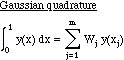Gaussian quadrature

To calculate the value of an integral one can approximate the function using a polynomial defined on subintervals. In Gaussian quadrature we choose as quadrature points the m roots to Pm(x) = 0. Call these points {xj}. Then the quadrature isThe quadrature is exact when y is a polynomial of degree 2m­1 in x. Because there are m weights and m Gauss points, we have 2m parameters which are chosen to exactly represent a polynomial of degree 2m-1, which has 2m parameters. The Gauss points and weights are given in the Table. The weights can either be defined with W(x) in the integrand as well.

Table. Gaussian quadrature points and weights
 N xi Wi 1 0.500000 00000 0.66666 66667 2 0.21132 48654 0.50000 00000 0.78867 51346 0.50000 00000 3 0.11270 16654 0.27777 77778 0.50000 00000 0.44444 44445 0.88729 83346 0.27777 77778 4 0.06943 18442 0.17392 74226 0.33000 94783 0.32607 25774 0.66999 05218 0.32607 25774 0.93056 81558 0.17392 74226 5 0.04691 00771 0.11846 34425 0.23076 53450 0.23931 43353 0.50000 00000 0.28444 44444 0.76923 46551 0.23931 43353 0.95308 99230 0.11846 34425

For a given N the quadrature points x2, x3, ..., xNP-1 are given above. x1 = 0, xNP = 1. For N=1, W1=W3=1/6 and for N2 W1 = WNP=0.